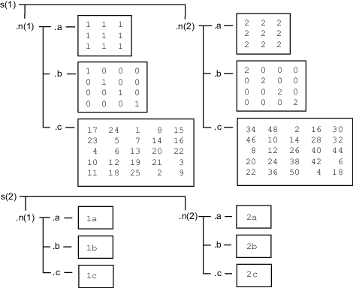## 访问嵌套结构体中的数据

`structName(index).nestedStructName(index).fieldName(indices)`

```s.n.a = ones(3); s.n.b = eye(4); s.n.c = magic(5);```

`third_row_b = s.n.b(3,:)`

```third_row_b = 0 0 1 0```

```s(1).n(2).a = 2*ones(3); s(1).n(2).b = 2*eye(4); s(1).n(2).c = 2*magic(5); s(2).n(1).a = '1a'; s(2).n(2).a = '2a'; s(2).n(1).b = '1b'; s(2).n(2).b = '2b'; s(2).n(1).c = '1c'; s(2).n(2).c = '2c';````part_two_eye = s(1).n(2).b(1:2,1:2)`

```part_two_eye = 2 0 0 2```